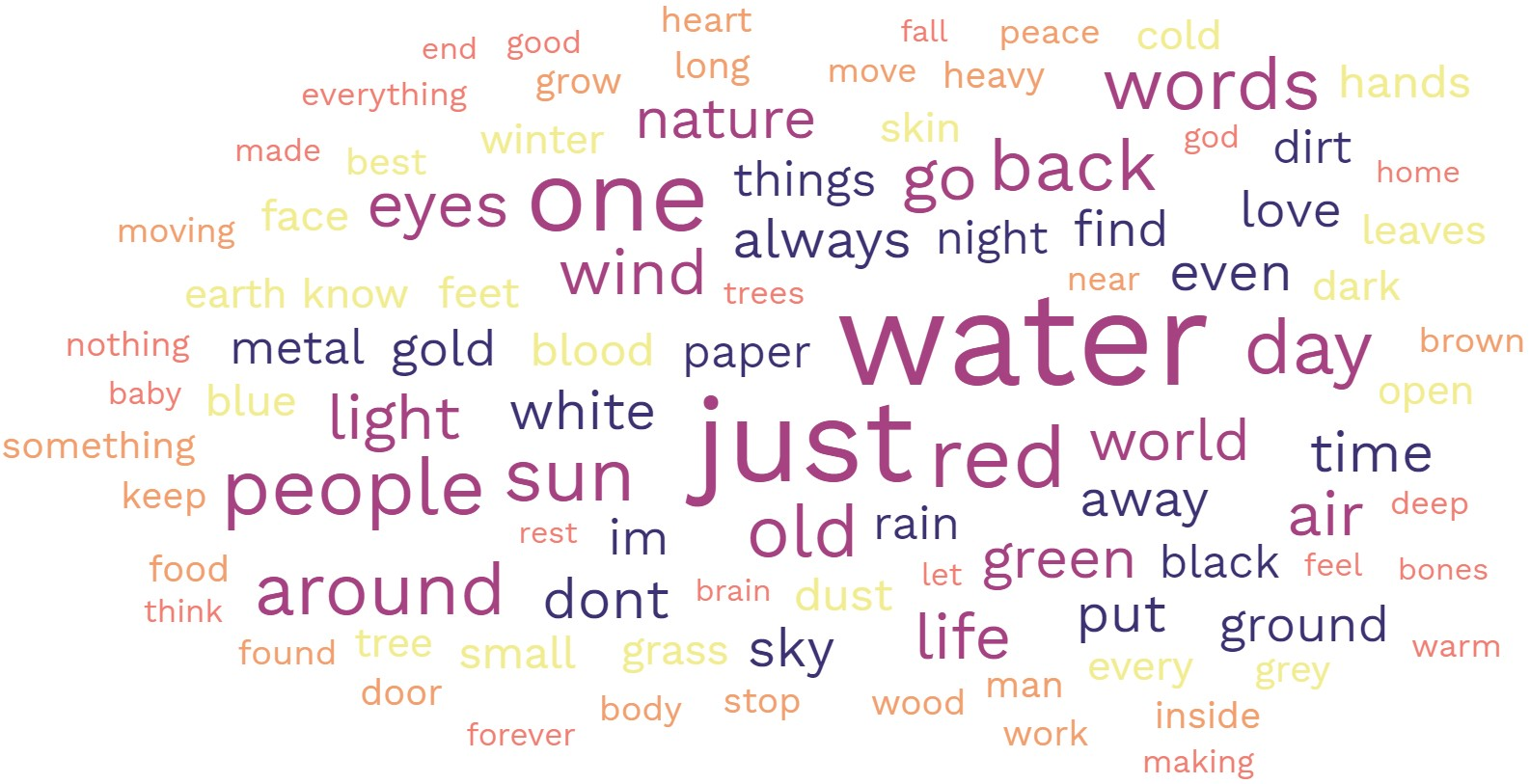# Experimental Poetry 8

7/11/19

I decided to do a found poem of sorts, but this time make it so the "poem" contents, including the titles, were made from the code that was used to create the poem. Talk about a poet spilling their guts.

I made a list of all the functions, variables, operations, and numbers used in the code in Excel to create this poem. Then I randomly sampled from this list, with replacement, to create the three following poems:

Enjoy!

### 4 A M P COUNTA I E Y ROW IF

```P ROW INT 3 LOOKUP RAND() 10 J SUM K
M INDEX W : ROUND B 9 P S >
CONCATENATE IF K C 10 > V W COUNTA Z
\$ <= 10 O 2 W N R RANDBETWEEN AND
) < LEN LOOKUP D W < * T A
\$ R O B G INDEX + M V >
A : PROPER L L X <= 3 8 =
0 3 D RAND() W 3 E RANDBETWEEN COUNTIF T
V W T H 6 RAND() PROPER RIGHT LARGE )
X W V 5 CONCATENATE ( LARGE 10 ( O
```

### D RIGHT + Q M LEN V K 7 N

```LEN RAND() INT E LARGE * L SUM + IF
LOOKUP J Z COUNTIF R 5 SUM 8 2 INT
ROUND F 3 8 ROUND 5 SUM AND 2 ROW
IF RIGHT A J K COUNTA Y INDEX 9 RIGHT
4 E 0 I 9 O RAND() ( Y ROW
MID X RANDBETWEEN COUNTA L - B O 10 7
4 MID D X ROW S W LARGE RAND() Q
SUM 3 N RAND() ROW F 3 9 0 10
4 COUNTA RIGHT O D * LOOKUP SUM H R
O T IF > ROUND INDEX < Z <= CONCATENATE
```

### LEN SUM M LEN RANDBETWEEN L Y MID LARGE O

```<= P 6 I INDEX 8 W INT 7 \$
MID R >= LOOKUP O \$ X ) SOUNDEX 7
SUM OR Y \$ * 10 >= Y V L
< T 2 R ( 2 > 4 5 -
SUM 2 T AND B COUNTIF RANDBETWEEN PROPER RAND() 8
T 0 \$ >= SOUNDEX RANDBETWEEN INT X >= M
M F 7 LARGE L INT <= Z CONCATENATE N
K F 9 5 S S G T B ROW
B T R \$ K < * J V >=
LOOKUP U H 9 I SOUNDEX S Q INDEX L
```

Ahh, computery beauty.

Please credit Statisticool.com if you use this idea.

Like:
Dislike:

For poetry, I recommend:

• High Quality Every Day Carry (EDC) Bolt Action Pens & Pencils by Bastion. Use discount code JS20 to get 20% off!
• Shop at The Poet Life store

Please show me a randomly selected poemIf you enjoyed any of my content, please consider supporting it in a variety of ways: